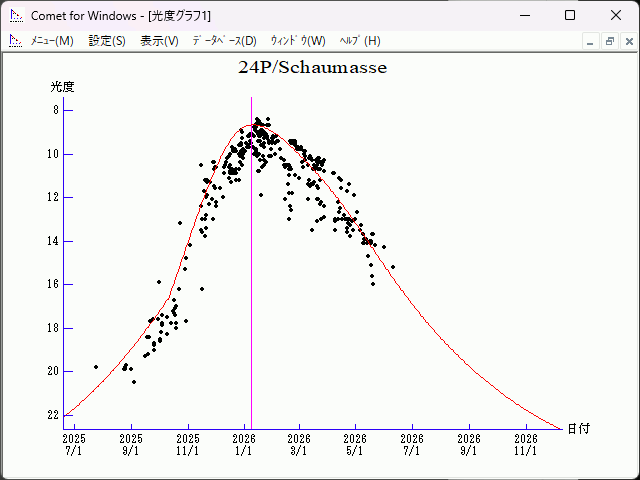# 24P/Schaumasse (2026)###Orbital Elements

```Epoch 2025 Dec. 31.0 TT = JDT 2461040.5
T 2026 Jan. 8.29008 TT                                  MPCW
q   1.1839534            (2000.0)            P               Q
n   0.12056074     Peri.   58.48171     -0.71166265     -0.67484743
a   4.0581876      Node    78.27274     +0.55778383     -0.71173805
e   0.7082556      Incl.   11.50179     +0.42709890     -0.19496125
P   8.18
From 1049 observations 1992 Sept. 24-2018 Apr. 28, mean residual 0".9.
Nongravitational parameters A1 = +0.06, A2 = -0.0477.
```

###Finding Charts###Magnitudes Graph

```        m1 = 12.0 + 5 log d + 17.0 log r  [   ,-90]  (             - 2025 Oct. 10)
m1 =  6.0 + 5 log d + 45.0 log r  [-90,  0]  (2025 Oct. 10 - 2026 Jan.  8)
m1 =  7.2 + 5 log d + 30.0 log r  [  0,170]  (2026 Jan.  8 - 2026 June 27)
m1 = 12.0 + 5 log d + 17.0 log r  [170,   ]  (2026 June 27 -             )
```##### The orbital elements are published on M.P.E.C. 2018-H105. The charts are made with StellaNavigator Ver.10 (AstroArts). The magnitudes graphs are made with Comet for Windows.